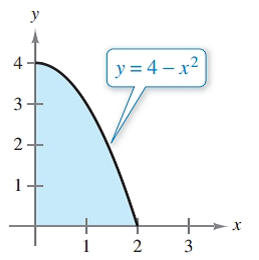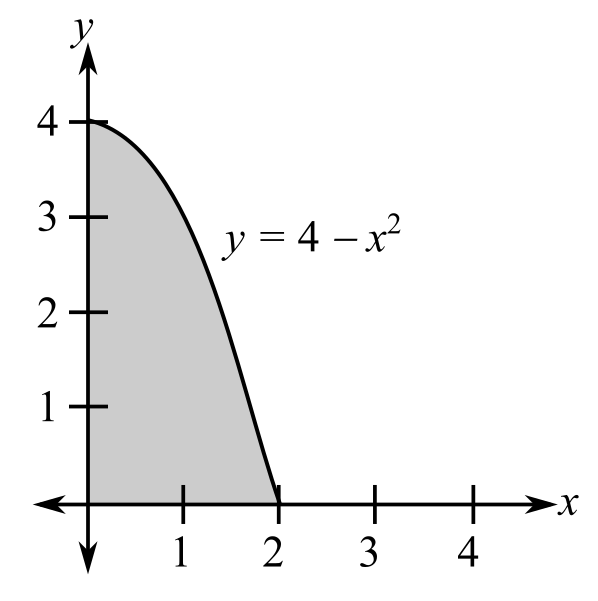Chapter 7.8, Problem 29E### Calculus: An Applied Approach (Min...

10th Edition
Ron Larson
ISBN: 9781305860919

#### Solutions

Chapter
Section### Calculus: An Applied Approach (Min...

10th Edition
Ron Larson
ISBN: 9781305860919
Textbook Problem
43 views

# Finding Area with a Double Integral In Exercises 25-30, use a double integral to find the area of the specified region. See Example 3.To determine

To calculate: The area of region by using double integration shown below.Explanation

Given Information:

The provided region is.

Formula used:

If a region is R defined in the domain of ayb and cxd, then,

The area of the region R is,

A=cdabdydx

Calculation:

Consider the region,

The bound for x are 0x2 and the bound for y are 0y4x2.

The area of the region is

A=0204x2dydx

Integrate with respect to y by holding x constant,

0204x2dydx=06[y]

### Still sussing out bartleby?

Check out a sample textbook solution.

See a sample solution

#### The Solution to Your Study Problems

Bartleby provides explanations to thousands of textbook problems written by our experts, many with advanced degrees!

Get Started

#### Sketch the graphs of the equations in Exercises 512. yx=1

Finite Mathematics and Applied Calculus (MindTap Course List)

#### let f(x) = x3 + 5, g(x) = x2 2, and h(x)= 2x + 4. Find the rule for each function. 2. f g

Applied Calculus for the Managerial, Life, and Social Sciences: A Brief Approach

#### The quadratic approximation for at a = 1 is:

Study Guide for Stewart's Single Variable Calculus: Early Transcendentals, 8th